Most Affordable JEE | NEET | 8,9,10 Preparation by Kota's Top IITian Doctor Faculties

# EMF & Internal Resistance of a cell | Terminal Potential Difference | Types of cells`
There are some components that are integral part of a circuit like EMF & Internal resistance of a cell and they are all inter-related. In this article we will explore about electrochemical cells, EMF and internal resistance of a cell. Later we will also see terminal potential difference and types of cell in detail. Contents:

## Electrochemical Cell

An electrochemical cell is a device capable of either generating electrical energy from chemical reactions or using electrical energy to cause chemical reactions. The electrochemical cells which generate an electric current are called voltaic cells or galvanic cells and those that generate chemical reactions, via electrolysis for example, are called electrolytic cells. A common example of a galvanic cell is a standard 1.5 volt cell meant for consumer use. A battery consists of one or more cells, connected either in parallel, series or series-and-parallel pattern. [source] An electrochemical cell is a device which by converting chemical energy into electrical energy maintains the flow of charge in a circuit. It usually consists of two electrodes of different materials and an electrolyte. The electrode at higher potential is called anode and the one at lower potential is cathode. [caption id="attachment_4602" align="aligncenter" width="177"]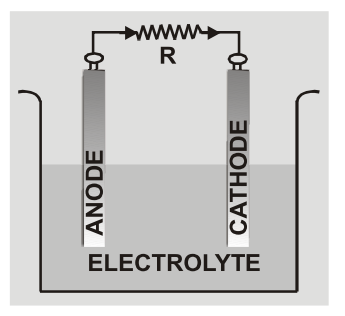Electrochemical Cell[/caption]

## Electromotive Force (EMF)

The emf of a cell is defined as work done by cell in moving a unit positive charge in the whole circuit including the cell once.
1. emf E = W/q; SI unit is joule/coulomb or volt.
2. emf is the maximum potential difference between the two electrodes of the cell when no current is drawn from the cell.
3. emf is the characteristic property of cell and depends on the nature of electrodes and electrolyte used in cell.
4. emf is independent of quantity of electrolyte, size of electrodes and distance between the electrodes.

## Internal Resistance of a Cell

The opposition offered by the electrolyte of the cell to the flow of electric current through it is called the internal resistance of the cell. The internal resistance of cell depends on.
1. Distance between electrodes (r ∝ d) larger is the separation between electrodes more is the length of electrolyte through which ions have to move so more is internal resistance.
2. Conductivity or nature of electrolyte (r ∝ 1/σ)
3. Concentration of electrolyte (r ∝ c)
4. Temperature of electrolyte (r ∝ 1/T)
5. Nature and area of electrodes dipped in electrolyte (r ∝ 1/A)

## Terminal Potential Difference

The potential difference between the two electrodes of a cell in a closed circuit i.e. when current is being drawn from the cell is called terminal potential difference.

### (a) When cell is Discharging

When cell is discharging current inside the cell is from cathode to anode. [caption id="attachment_4603" align="aligncenter" width="230"]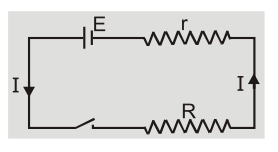Cell is discharging[/caption] Current ${\rm{I}} = {{\rm{E}} \over {{\rm{r}} + {\rm{R}}}}$ or $\quad {\rm{E}} = {\rm{IR}} + {\rm{Ir}} = {\rm{V}} +$ Ir $\quad$ or ${\rm{V}} = {\rm{E}} -$ Ir When current is drawn from the cell potential difference is less than emf of cell. Greater is the current drawn from the cell smaller is the terminal voltage. When a large current is drawn from a cell its terminal voltage is reduced.

### (b) When cell is Charging

When cells are charging current inside the cell is from anode to cathode. Current ${\rm{I}} = {{{\rm{V}} - {\rm{E}}} \over {\rm{r}}}\quad$ or $\quad {\rm{V}} = {\rm{E}} + {\rm{Ir}}$ During charging terminal potential difference is greater than emf of cell. [caption id="attachment_4604" align="aligncenter" width="234"]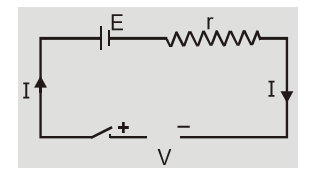Cell is charging[/caption]

### (c) When cell is in Open circuit

In open circuit, ${\rm{R}} = \infty$ ${\rm{I}} = {{\rm{E}} \over {{\rm{R}} + {\rm{r}}}} = 0$ So, ${\rm{V}} = {\rm{E}}$ In open circuit terminal potential difference is equal to emf and is the maximum potential difference which a cell can provide.

### (d) When cell is Short-circuited

In short circuit $R = 0$ so $I = {E \over {R + r}} = {E \over r}\quad$ and $\quad V = IR = 0$ In short circuit current from cell is maximum and terminal potential difference is zero.

### (e) Power transferred to load by cell

${\rm{P}} = {{\rm{I}}^2}{\rm{R}} = {{{{\rm{E}}^2}{\rm{R}}} \over {{{({\rm{r}} + {\rm{R}})}^2}}}$ $\matrix{{{\rm{so}}} & {{\rm{P}} = {{\rm{P}}_{\max }}\quad {\rm{ if }}\quad {{{\rm{dP}}} \over {{\rm{dR}}}} = 0} \cr{\rm{P}} & { ={{\rm{P}}_{\max }}\quad {\rm{ if }}\quad {\rm{r}} = {\rm{R}}} \cr}$ Power transferred by cell to load is maximum when $r = R$ and ${P_{\max }} = {{{E^2}} \over {4r}} = {{{E^2}} \over {4R}}$ [caption id="attachment_4605" align="aligncenter" width="243"]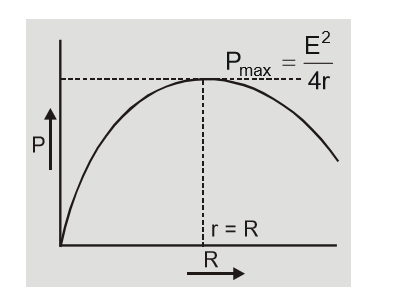Power transferred to load by cell[/caption]

## Difference between EMF and Potential Difference

[caption id="attachment_4606" align="aligncenter" width="594"]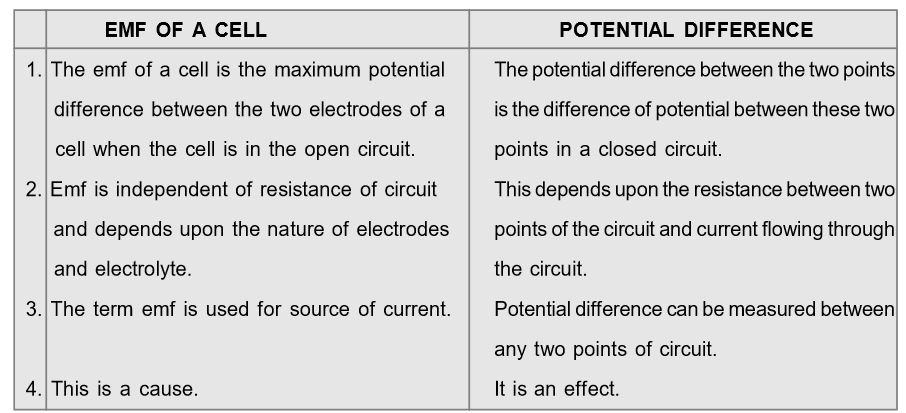Difference between EMF and Potential Difference[/caption]

1. The current inside a cell is due to motion of both positive and negative ions while outside it depends on nature of circuit elements like conductors, semiconductor, gas or electrolyte.
2. The cell is a source of constant emf and not of constant current because if resistance of circuit changes then current I = E/r + R also changes but emf remains constant.
3. As I = E/r so more current can be drawn from a cell with larger emf and smaller internal resistance. Example: In lead acid accumulator E = 2.05 V and rmin = 0.1Ω.
4. With use of cell its internal resistance increases appreciably but emf fall slightly. The current delivering ability is reduced.
5. A cell neither creates nor destroys charge but maintains the flow of charge by providing required energy.
6. The emf of cell is taken to be positive in a circuit if current inside a cell is from negative to positive i.e. during discharging otherwise negative. [caption id="attachment_4607" align="aligncenter" width="594"]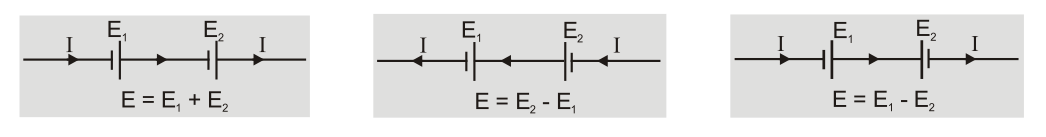EMF of a cell[/caption]
7. Capacity of a battery is equal to product of current in ampere and time in hour for which a cell can operate. It depends on the amount of electrolyte and size of cell. Example: capacity 8Ah means we can draw 8A current for one hour or 2A current for 4 hours.

## Type of Cells

### Primary Cells

The cells which cannot be recharged electrically are called primary cells. Here the original state of cell cannot be brought back by passing electrical energy through cell from external source after cell is discharged. Example: Voltaic cell, Daniel cell, Leclanche cell, Manganese-alkaline cell, Mercury button cell etc.

### Secondary Cells

The cells in which chemical process is reversible are called secondary cells. Here original chemical state of cell can be brought back by passing electrical energy through cell from external source. Example: Lead acid accumulator, alkali cells etc.

### Comparative Study of Various Cells

[caption id="attachment_4701" align="aligncenter" width="571"]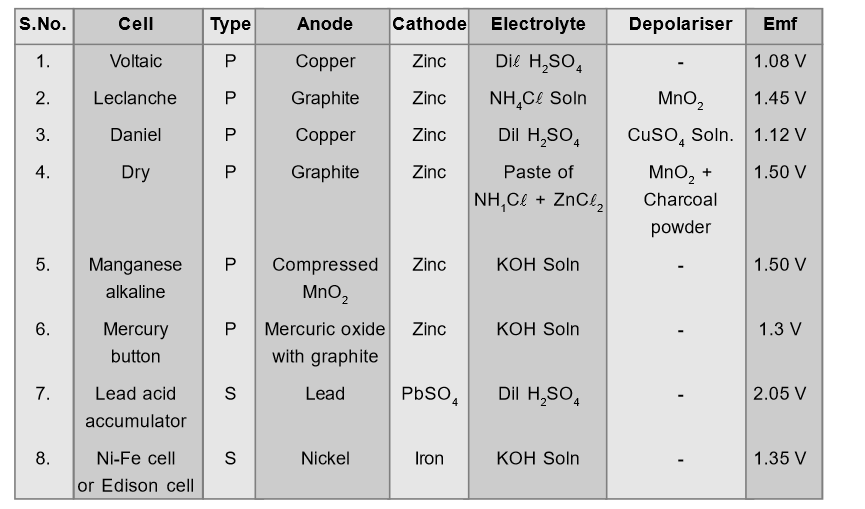Comparative Study of Various Cells[/caption]

### DEFECTS OF VOLTAIC CELL

Local Action: This is due to impurities of copper, iron carbon etc. in commercial zinc. When zinc rod is dipped in electrolyte the impurities and zinc in contact form small local cells in which small currents are produced resulting in wastage of zinc even when cell is not being used. This defect is called local action. This is overcome by amalgamating zinc rod by mercury. When cell is being used fresh zinc continues to come on surface so chemical reaction continues local action. Polarization: Polarization is formation of hydrogen gas bubbles on the anode of cell. This causes an increase in internal resistance because layer of Hydrogen is a bad conductor. The hydrogen ions moving towards anode are unable to reach anode and transfer their charge. These positively charged ions set up a field from hydrogen to zinc resulting in back emf weakening the action of cell. This defect may be overcome by using a depolarizer i.e. oxidising agent like MnO$_2$ or CuSO$_2$ which oxidizes hydrogen to water. The construction of some cells is shown below: [caption id="attachment_4702" align="aligncenter" width="541"]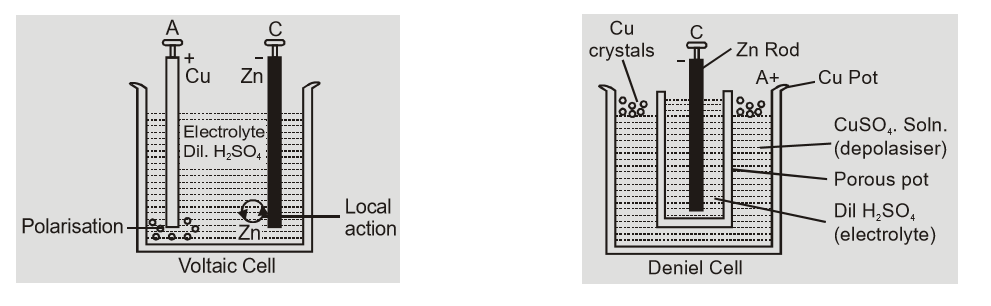Construction of Voltaic and Daniel cells[/caption] [caption id="attachment_4704" align="aligncenter" width="529"]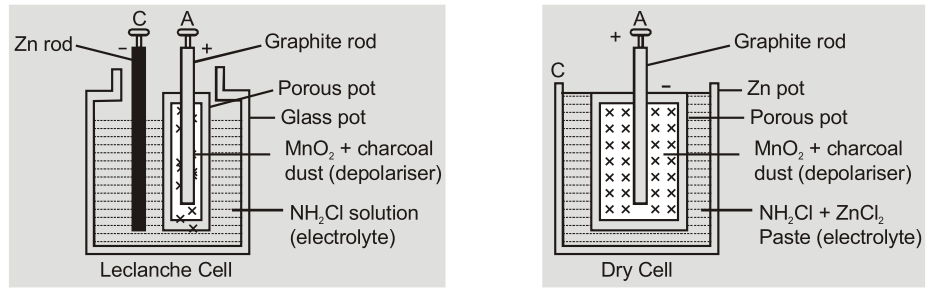Construction of Leclanche and Dry cells[/caption]     Also Read: